Next: 5.1.3 Factor Interpretation Up: 5.1 Statistical Analysis of Previous: 5.1.1 Mathematical Background and

## 5.1.2 Factor Extraction

The factors are extracted based on the fundamental theorem of factor analysis , which says, that every observed value can be written as a linear combination of hypothetical factors. The base of factors is chosen in a way that the base vector is an element which is most responsible for occurring variances. The components are sorted according to their contribution to the variance. Thus, PCA is primarily a method to simplify data sets and only at a second stage a means to extract physically interpretable quantities.

For the reduced example two factors are considered. The criterion for the chosen number of factors in the extraction is an Eigenvalue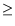1, as can be seen in the so-called Scree plot in Fig. 5.3, which is a plot of Eigenvalue vs. factor number .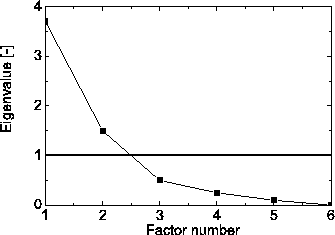Extracting the factors via SPSS it is found that the first extracted factor accounts for 62% of the occurring variance, while the second factor accounts for an additional 25 %. This leads to an overall 87% explanation of the variance. The resulting factor matrix is found in Table 5.1.

Table 5.1: Final statistics: factor matrix.
 Variable Factor 1 Factor 2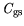0.91 -0.284 Gate source capacitance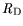-0.882 0.429 Drain resistance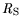-0.868 0.441 Source resistance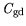0.822 0.432 Gate drain capacitance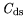0.731 0.50 Drain-source capacitance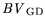-0.369 -0.8 Gate drain breakdown voltage

A similar quantity as Factor 1 has been extracted in  for InAlAs/InGaAs HEMTs. The physical explanation was attributed to variations of doping during MBE growth, however, also mechanisms due to the semiconductor process are likely, as explained in the next section.Next: 5.1.3 Factor Interpretation Up: 5.1 Statistical Analysis of Previous: 5.1.1 Mathematical Background and
Quay
2001-12-21